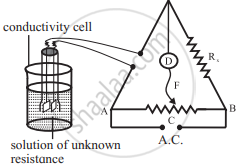Advertisement Remove all ads

# Answer the following in one or two sentences. What is a cell constant ? - Chemistry

Answer in Brief

What is a cell constant? What are its units? How is it determined experimentally?

Advertisement Remove all ads

#### Solution

For a given cell, the ratio of separation (l) between the two electrodes divided by the area of cross-section (a) of the electrode is called the cell constant.

Cell constant = "l"/"a"

The unit of cell constant is m-1 (SI unit) or cm-1 (C.G.S unit).1) The cell constant is determined using the 1 M, 0.1 M or 0.01 M KCl solutions. The conductivity of KCl solution is well tabulated at various temperatures.

2) The resistance of KCl solution is measured by Wheatstone bridge as shown in the figure.

3) AB is the uniform wire. Rx is the variable known resistance placed in one arm of Wheatstone bridge. The conductivity cell containing KCl solution of unknown resistance is placed in the other arm of Wheatstone bridge.

4) D is a current detector. F is the sliding contact that moves along AB. A.C. represents the source of alternating current.

5) The sliding contact is moved along AB until no current flows. The detector D shows no deflection. The null point is, thus, obtained at C.

6) According to Wheatstone bridge principle,

("R"_(("solution")))/(l("AC")) = "R"_"x"/(l("BC"))

Hence, "R"_(("solution")) = (l("AC"))/(l("BC")) xx "R"_"x"

7) By measuring lengths AC and BC and knowing Rx , resistance of KCl solution can be calculated.

8) The cell constant is given by

Cell constant = kKCl × "R"_(("solution"))

The conductivity of KCl solution is known. The cell constant, thus, can be calculated.

Is there an error in this question or solution?
Advertisement Remove all ads

#### APPEARS IN

Balbharati Chemistry 12th Standard HSC for Maharashtra State Board
Chapter 5 Electrochemistry
Exercise | Q 2.01 | Page 118
Balbharati Chemistry 12th Standard HSC for Maharashtra State Board
Chapter 5 Electrochemistry
Exercise | Q 4.07 | Page 119
Advertisement Remove all ads
Advertisement Remove all ads
Share
Notifications

View all notifications

Forgot password?